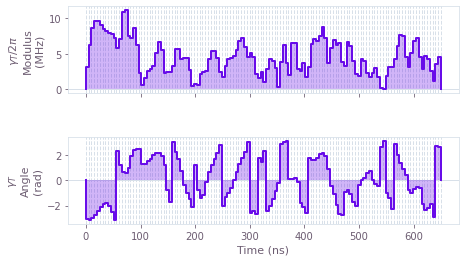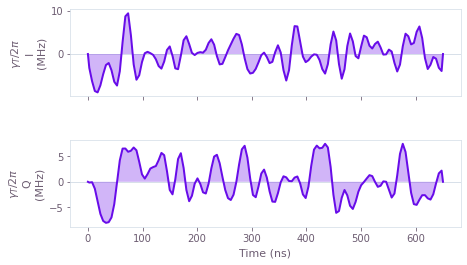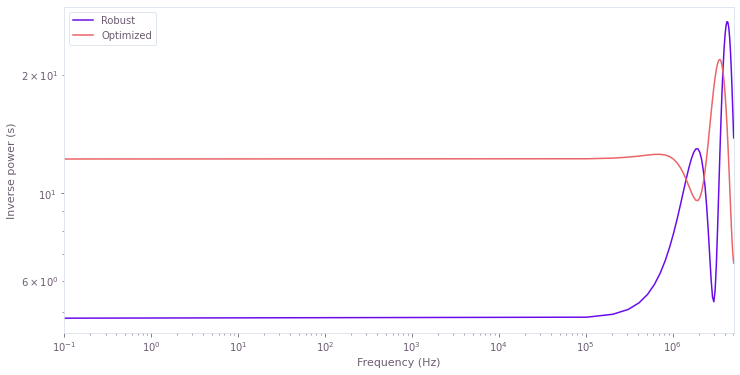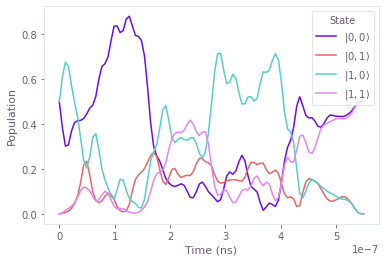# How to visualize data with the Q-CTRL Visualizer package

Display quantum controls and dynamics

The Q-CTRL Visualizer package offers a range of functions and options to help you implement and understand quantum control and dynamics. The functions are easily applied to outputs from the Boulder Opal tools for quantum control, and support the visualization of various use-cases:

• control pulses,
• control robustness,
• quantum dynamics, and
• implementing Q-CTRL styling in Matplotlib.

## Visualization overview

Here we present the different visualization functions and options that you can use to display different characteristics of your quantum system.

### Control pulses

The Q-CTRL Visualizer package offers tools to visualize controls of different forms.

The plot_controls function is a flexible way to display controls, and it is routinely used in our documentation to display optimized control outputs. The required inputs are the matplotlib Figure which will contain the plots, often specified as figure=plt.figure(), and the dictionary of controls to be plotted. Dictionary keys should be the names of the controls, where each control contains the pulse segment durations and values as specified in the reference documentation. A number of convenience options can help tailor the display to particular use-cases:

• use the polar option to specify whether to display the controls in polar coordinates,
• use the smooth option to display linear interpolation between pulse samples rather than piecewise-constant segments,
• select your desired unit_symbol string to replace the Hz default, and
• specify whether control values should be divided by a two_pi_factor in the plots.

The plot_smooth_controls function is similar to plot_controls, but displays controls given in terms of pulse sample times (in units of seconds), and values. As above, the polar, unit_symbol (to specify the units for the control values), and two_pi_factor options can tailor the control display.

For dynamical decoupling sequences, such as those available from Q-CTRL Open Controls, plot_sequences can be used to display the controls. As for the other control plots, the figure (matplotlib Figure) must be provided, along with the seq dictionary of controls. This dictionary is similar to those for plot_control, but takes offset instead of duration, and rotation instead of value, as described in the reference documentation.

### Control robustness

Filter functions are useful tools to quantify the control robustness to given noise sources. To calculate filter functions, refer to our user guide: How to calculate and use filter functions for arbitrary controls. Once calculated, you can visualize the filter functions with plot_filter_functions from the Q-CTRL Visualizer. The figure (matplotlib Figure to contain the plots) should be provided, along with the filter_functions dictionary. Here the keys are the names of the filter functions, and the values are the list of samples for the given filter function. Each sample is a dictionary with the keys:

• the frequency (in Hz) at which the sample was taken,
• the inverse_power (in seconds) for the filter function value at the sample, and
• the (optional) inverse_power_uncertainty (in seconds).

### Quantum dynamics

The Q-CTRL Visualizer also provides functions to visualize the state evolution, which helps understand the system dynamics. These Bloch sphere visualizers require IPython and must be run from a Jupyter notebook.

For a single qubit, there are several options. The display_bloch_sphere function takes a [T,2]-shaped array of pure states and shows the state trajectory on a Bloch sphere over the T trajectory steps. Similarly, the function display_bloch_sphere_from_density_matrices takes the [T,2,2]-shaped array of density matrices to show the state trajectory for pure or mixed states, such that the trajectory points lie on the surface of the Bloch sphere or inside it, respectively. The Bloch sphere trajectory can also be constructed using the [T,3]-shaped array of Cartesian Bloch-sphere coordinates with display_bloch_sphere_from_bloch_vectors, which can represent both mixed and pure states. Options for the Bloch sphere display are available in the reference documentation.

For two qubits, the display_bloch_sphere_and_correlations function provides a visualization of Bloch spheres for each qubit as well as three tetrahedra to represent the correlations between qubit observables, with axes representing covariances $V(A\otimes B)$ for Pauli operators $A,B \in \{\sigma_X, \sigma_Y, \sigma_Z\}$. Additionally, there is a bar displaying the concurrence to quantify entanglement. The required input is a [T,4]-shaped array representing the T trajectory steps of the 4-dimensional pure state.

### Implementing Q-CTRL styling in Matplotlib

It is possible to apply Q-CTRL styling across plots generated using matplotlib, by implementing the get_qctrl_style function as follows:

import matplotlib.pyplot as plt
from qctrlvisualizer import get_qctrl_style
plt.style.use(get_qctrl_style())

This produces plot styles that are consistent with our inbuilt plotting functions, as shown in our documentation such as the application note 'Demonstrating SU(3) gates on superconducting hardware'.

## Worked example: Visualizing two-qubit optimization results and dynamics

To demonstrate the usage of the visualization functions, we consider a two-qubit system described by the following Hamiltonian:

$$H = \chi a^\dagger a b^\dagger b + \left(\gamma_T (t) b + H.c.\right) + \left(\gamma_C (t) a + H.c.\right),$$

which can represent two-level treatment of a transmon system coupled to a cavity in the dispersive regime, as explored in the application note 'Performing optimal Fock state generation in superconducting resonators'. Here $\chi$ is the dispersive shift, $a$ and $b$ are the annihilation operators of the cavity and transmon systems, respectively, and $\gamma_{T(C)}(t)$ is the complex drive amplitude applied to the transmon (cavity) qubit.

We consider the case that smooth controls have been optimized (as in the application note) to perform a target two-qubit CX operation. This optimization was performed both with and without robustness to fluctuations in the amplitude of the transmon control pulse. Note that the optimization procedure and robust optimization are both introduced in our user guides.

In the following cell we import the relevant packages and functions.

import jsonpickle
import matplotlib.pyplot as plt
import numpy as np
from attr import asdict
from qctrlvisualizer import (
display_bloch_spheres_and_correlations,
get_qctrl_style,
plot_controls,
plot_filter_functions,
)

plt.style.use(get_qctrl_style())

# Predefined pulse imports
import qctrlopencontrols

from qctrl import Qctrl

# Starting a session with the API
qctrl = Qctrl()

# Return a variable from a json file
file_path = "./resources/visualize-data/"
f = open(file_path + file_name, "r+")
decoded = jsonpickle.decode(encoded)
f.close()
return decoded


We next import the result of qctrl.functions.calculate_optimization, where we specified the drive signal names (\$\gamma_T\\$, \$\gamma_C\\$") among the output_node_names arguments. We can now plot these control pulses directly, using the plot_controls function. As an example, we plot the robust control on the transmon qubit below.

robust_result = load_var("robust_control_result")

controls = {
"$\gamma_T$": robust_result.output["$\gamma_T$"],
}
plot_controls(plt.figure(), controls, polar=True)For a smooth representation of the I and Q transmon control pulse components, we adjust the plotting options as shown below.

plot_controls(plt.figure(), controls, polar=False, smooth=True)We next visualize the robustness of the controls to noise in the transmon drive amplitude. Robustness can be quantified using filter functions, as demonstrated in the user guide 'How to calculate and use filter functions for arbitrary controls'. In the cell below, we import results from the qctrl.functions.calculate_filter_function function applied to the optimized and robust controls, with respect to multiplicative amplitude fluctuation noise on the transmon drive. We then display the filter functions using plot_filter_functions from the Q-CTRL Visualizer.

optimized_ff = load_var("optimized_filter_function")

plot_filter_functions(
plt.figure(),
{
"Robust": map(asdict, robust_ff.samples),
"Optimized": map(asdict, optimized_ff.samples),
},
)We can also display the quantum dynamics induced by the controls. In the cell below, we import the result of system simulation that followed the procedure in the user guide 'How to simulate quantum dynamics for noiseless systems using graphs'. We simulated the system dynamics with the optimized control pulses, and obtained the unitary evolution operators for 101 sample_times using qctrl.operations.time_evolution_operators_pwc. These unitaries acted on the initial state $H |0\rangle \otimes |0\rangle$. As in the user guide, the state at each of the sample times was returned by the qctrl.functions.calculate_graph function by referencing the states in the output_node_names argument.

To display the Bloch sphere dynamics of the system evolution, we first reshape the state data to the required [T, 4] dimensions. We then use the function display_bloch_sphere_and_correlations to display the dynamics. Note that the concurrence entanglement measure (the central bar) displays maximal entanglement after the operation; the ideal target CX operator indeed produces a two-qubit GHZ state when acted on the given initial state.

optimized_states = load_var("optimized_state").output["states"]["value"].squeeze()

display_bloch_spheres_and_correlations(optimized_states)


Next we display the state population dynamics to demonstrate the effect of the get_qctrl_style function on standard Matplotlib usage. Here the $|i,j\rangle$ state refers to the $i$ transmon qubit state and the $j$ cavity qubit state.

sample_times = np.linspace(0, 550e-9, len(optimized_states))
populations = np.abs(optimized_states.T) ** 2

fig, ax = plt.subplots()
ax.plot(sample_times, populations, label=r"$|0,0\rangle$")
ax.plot(sample_times, populations, label=r"$|0,1\rangle$")
ax.plot(sample_times, populations, label=r"$|1,0\rangle$")
ax.plot(sample_times, populations, label=r"$|1,1\rangle$")
ax.set_xlabel("Time (ns)")
ax.set_ylabel("Population")
plt.legend(title="State")
plt.show()